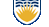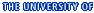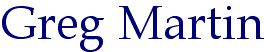ProfessorDepartment of Mathematics

I am the proud recipient of two Lester R. Ford Awards, in 2002 and 2007. These awards, established in 1964, are given by the Mathematical Association of America for “articles of expository excellence published in the American Mathematical Monthly”. The awards are named for Lester R. Ford, Sr., a distinguished mathematician, editor of the American Mathematical Monthly, 1942-46, and president of the MAA, 1947-48.

For the first award, I was cited for the paper “Absolutely Abnormal Numbers”, Amer. Math. Monthly 108 (October 2001), pp. 746-754. The citation read:

 Normal numbers are those whose decimal (or other base) expansions contain all possible finite configurations of digits with roughly their expected frequencies. It is known that almost all real numbers are normal to any given base. Thus, the set of absolutely abnormal numbers, those that are not normal to any base, must be a set of measure zero. The rational numbers provide easy examples of such numbers. However, the set of absolutely abnormal numbers is an uncountable dense subset of the real numbers, hence must contain irrational numbers. This article is unique in that the author actually constructs an example of an irrational absolutely abnormal number. In an exceptionally clear, straightforward fashion the reader is shown the motivation for considering Liouville numbers, followed by the description of one particular such number, and finally the main result, that this number is absolutely abnormal. Moreover, as pointed out by the author, this technique can be generalized to give uncountably many irrational absolutely abnormal numbers. This paper provides some very satisfying results in an area of mathematics where one can prove the existence of some types of numbers but actual constructions are rare.

For the second award, I was cited for the paper “Prime number races”, Amer. Math. Monthly 113 (January 2006), pp. 1-33. The citation read:

 The races in this exciting article are between primes in different congruence classes mod q. That is, fix q and consider for varying values of integers a relatively prime to q the functions πq,a(x) = the number of primes of the form qn+a less than or equal to x. The prime number theorem for arithmetic progressions tells us that, asymptotically, the results for different such a will be the same, but this does not address whether or how often πq,a(x) > πq,b(x) for specific values of x. Granville and Martin offer a wonderful array of refined results about πq,a(x) vs. πq,b(x), beginning with Chebychev's observation that π4,3(x) appears to be larger than π4,1(x), coupled with J. E. Littlewood's 1914 theorem that there are arbitrarily large values of x for which π4,1(x) > π4,3(x). In addition, the authors highlight M. Rubinstein and P. Sarnark's amazing 1994 result that, as X → ∞, 1/ln X × Σ { 1/x: x ≤ X, π4,3(x) > π4,1(x) } → .9959... Of course, the generalized Riemann hypothesis figues in the discussion, as does a survey of some very recent research including that of both authors, as well as of teams of graduate and undergraduate (!) students.